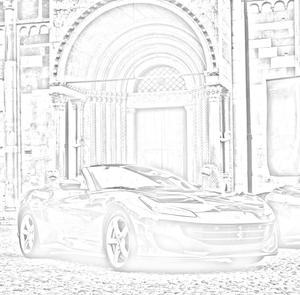# Convert Image into Sketch

In Python an image is just a two dimensional array of integers. So one can do a couple of matrix manipulations using various python modules in order to get some very interesting effects. In order to convert normal image to sketch we will change it’s original RGB values and assign its RGB values similar to grey, in this way a sketch of the input image will be generated.

### Approach:

1. Import all required modules (numpy, imageio, scipy.ndimage, OpenCV)
2. Take Image input
3. Check RGB value of image and convert into according RGB values
4. Show finale image output using cv2.imwrite()

## Python3

 `# Python program to Convert Image into sketch ` ` `  ` `  `# import all the required modules ` `import` `numpy as np ` `import` `imageio ` `import` `scipy.ndimage ` `import` `cv2 ` ` `  ` `  `# take image input and assign variable to it ` `img ``=` `"4.jpeg"` ` `  ` `  `# function to convert image into sketch ` `def` `rgb2gray(rgb): ` `    ``# 2 dimensional array to convert image to sketch ` `    ``return` `np.dot(rgb[..., :``3``], [``0.2989``, ``0.5870``, .``1140``]) ` ` `  ` `  `def` `dodge(front, back): ` ` `  `    ``# if image is greater than 255 (which is not possible) it will convert it to 255 ` `    ``final_sketch ``=` `front``*``255``/``(``255``-``back) ` `    ``final_sketch[final_sketch > ``255``] ``=` `255` `    ``final_sketch[back ``=``=` `255``] ``=` `255` ` `  `    ``# to convert any suitable existing column to categorical type we will use apect function ` `    ``# and uint8 is for 8-bit signed integer ` `    ``return` `final_sketch.astype(``'uint8'``) ` ` `  ` `  `ss ``=` `imageio.imread(img) ` `gray ``=` `rgb2gray(ss) ` ` `  `i ``=` `255``-``gray ` ` `  ` `  `# to convert into a blur image ` `blur ``=` `scipy.ndimage.filters.gaussian_filter(i, sigma``=``13``) ` ` `  ` `  `# calling the fuction ` `r ``=` `dodge(blur, gray) ` ` `  ` `  `cv2.imwrite(``'4.png'``, r)`

#### Example 1:

Input image:Output:#### Example 2:

Input image:Output:My Personal Notes arrow_drop_up- Computer Science and Engineering student - Coder

If you like GeeksforGeeks and would like to contribute, you can also write an article using contribute.geeksforgeeks.org or mail your article to contribute@geeksforgeeks.org. See your article appearing on the GeeksforGeeks main page and help other Geeks.

Please Improve this article if you find anything incorrect by clicking on the "Improve Article" button below.

Article Tags :
Practice Tags :

Be the First to upvote.

Please write to us at contribute@geeksforgeeks.org to report any issue with the above content.